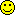Significance of Correlation CoefficientsMessage/AuthorClaudiaB posted on Monday, April 08, 2013 - 7:38 am
Hi,

significance of correlation coefficients is usually only dependent on the N of the sample and the correlation coefficient r.

I ran a correlation model in Mplus and there the significances of the correlation coefficients (WITH) are calculated basing on the ratio of the coefficient to its standard error.

In my data I get the following:

Estimate S.E. Est./S.E. P-Value
A WITH B -0.300 0.088 -3.413 0.001

C WITH B 0.293 0.078 3.766 0.000

Thus the strongest correlation (.30) is less significant than the smaller one (.29), which in the usual way of calculating significances would not be possible.

Is there some way to get "normal" significance values in Mplus? Or do you have some literature I could refer to when reporting significances as calcultated in Mplus?

Thank youLinda K. Muthen posted on Monday, April 08, 2013 - 11:55 am
Please send the output and your license number to support@statmodel.com so we can see the full situation.Linda K. Muthen posted on Tuesday, April 09, 2013 - 9:28 am
You are right that there is a simple formula for the standard error for a correlation that depends on only the correlation coefficient value and the sample size. But that simple formula is for a correlation among two observed variables that are normally distributed and where the variables are not part of an over-identified H0 model as in SEM. The correlations you point to involve latent variables and a categorical observed variable which are part of an over-identified H0 model estimated by WLSMV. In that case, the simple formula is not applicable. Instead, standard errors are computed using WLSMV. Their sizes depend on many factors including the model structure in complex ways.Andrea M Reina Tamayo posted on Monday, March 26, 2018 - 3:50 pm
I have a question about the correlation of type=threelevel.

I am interested in the within level correlations. I have run different models with different predictors and I noticed in the correlation matrix from SAMPSTAT that the correlations change as well as I add parameters in my model. I do not understand why this happens. Could you explain to me, how Mplus calculates the correlation coefficients?

In other programs, the correlations remain always the same even if there are more parameters in the matrices because they are bivariate correlations, why do they actually change in Mplus? for example the correlation between x2 and x1 is .23, but if I add more parameters to the model, the bivariate correlation between x2 and x1 changes to .03. It is not results from the model, but from SAMPSTAT. I find this a bit strange.Tihomir Asparouhov posted on Monday, March 26, 2018 - 5:18 pm
Probably you have to send your question to support@statmodel.com for a more precise answer.

In principle changes made to the estimated H0 model can not affect sampstat (the H1 model). The H1 model and the H0 model are estimated separately (see tech8 / log-likelihood values). So the changes you are making are affecting the definition of the H1 model. The two most common reasons are as follows:

1. Changing the status of a variable (i.e. on what level it is or if it is being centered or not). Doing something like that will channel correlation from one level to another.

2. Missing data - if you have a variable that is dependent in one model but independent in another affects how missing data is treated (missing data for covariates is deleted while missing data is modeled).Andrea M Reina Tamayo posted on Wednesday, March 28, 2018 - 2:45 am
Hi Tihomir,

Thank you for your response! It was helpful for sure, and my was option 2AndreaBack to top
Add Your Message HerePost:
Username: Posting Information:
This is a private posting area. Only registered users and moderators may post messages here.
Password:
Options: Enable HTML code in message
Automatically activate URLs in message
Action:Topics | Tree View | Search | Help/Instructions | Program Credits Administration